Function: splitup - break up an integral, sum or product

Calling Sequence:

splitup(f, a);

splitup(f, a, vflag);

splitup(f, x = a);

splitup(f, x = a, vflag);

Parameters:

f - an expression, usually containing at least one unevaluated definite integral, sum, or product

a - a value for the dummy or index variable of the int, sum or product

x - a dummy or index variable name (possibly indexed)

vflag - (optional) true or false.

Description:

Splits up an integral, sum or product at the value a of the dummy variable, separating the values of the dummy variable < a and those >= a into separate integrals, sums or products. Thus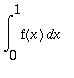becomes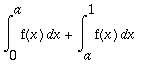and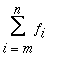becomes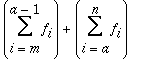.

The inert forms Int , Sum , Product may be used instead of int , sum , or product to ensure that the integral, sum or product is not evaluated before being split.

If the variable name x is specified, only those integrals, sums or products with dummy variable named x will be split. Otherwise, for nested integrals, sums or products, the outermost one is split.

No splitting is done if it is known that the value a is outside the interval of the integral, sum or product. Note that the result is still valid even if a is outside the interval, according to the conventions Maple uses: e.g. for a sum, if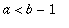,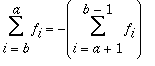.

The value a need not be numeric, but in the case of a sum or product, if it is numeric it must be an integer.

For a sum or product, unless the optional third argument vflag is false , if in any of the results of the split the difference between the bounds is an integer, that part is evaluated explicitly (even though it is a Sum or Product ). Thus if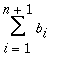is split at n the result would be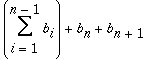. If vflag is false this would be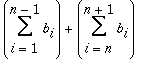. In the case of Int , no evaluation is done. The non-inert forms sum , product and int will always attempt to evaluate.

splitup is automatically mapped into arrays, lists, sets, functions, +, * and ^.

This function is part of the Maple Advisor Database library, and must be loaded before use by the command readlib(splitup); .

Examples:

> splitup(Int(f(x),x=a..c), b);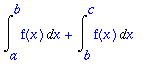> splitup(Sum(b[i], i=1 .. infinity) - Sum(b[i], i = 3 .. infinity), i = 3);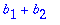> splitup(Sum(Product(c[i,j], i=k .. n), j = 1 .. m), i = p);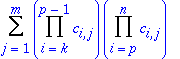See also: Int , Sum , Product , combine , assume , interface

Maple Advisor Database R. Israel, 1997